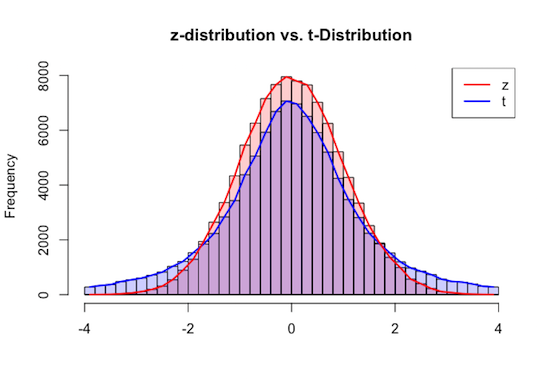R Project: The Rise of the t-Distribution

Consider the distribution of $z$-values given by the below formula, when resulting from a large group of samples of size $n$ taken from a population with mean $\mu$ and standard deviation $\sigma$ and where the distribution of sample means is close to normal.

$$z = \frac{\overline{x}-\mu}{\sigma/\sqrt{n}}$$

Given that the distribution of sample means is close to normal, we expect the distribution of the $z$-scores above to visually look almost identical to a standard normal distribution.

Of course in practice, we frequently don't know with any surety what the standard deviation of the population being sampled might be.

To this end, we wonder if and how this distribution might change if we estimate $\sigma$ with $s$, its sample-based approximation. That is to say, we wonder about the nature of the distribution of the $t$-values whose formula is given by the following:

$$t = \frac{\overline{x}-\mu}{s/\sqrt{n}}$$

To determine what happens, let's simulate this scenario in R.

First, create a function t.and.z.from.sample(sample, mu, sigma) that takes as arguments, a numerical vector representing our sample, the mean $\mu$ of the population from which the sample was drawn, and the standard deviation $\sigma$ of that same population. This function should return a vector of length 2, where the first element of that vector is the $t$ test statistic associated with that sample, and the second element of that vector is the $z$ test statistic associated with that sample.

Now use this function to create a function show.distributions(population, num.samples, sample.size, LB, UB, num.classes) that will draw two curves that approximate the shapes of the histograms of the $z$ values and of the $t$ values associated with some number of randomly selected samples of a given sample size from a given population. The function should also return a vector giving the standard deviation of the $t$-values and $z$-values found, respectively, as well.

An example is shown below. Note the population provided was a normal one, ensuring that the distribution of sample means by the Central Limit Theorem - even when the sample size is 3 - is normal.

> pop = rnorm(10000,60,12)
> show.distributions(pop,num.samples=100000,sample.size=3,LB=-4,UB=4,num.classes=30)
 3.8557808 0.9980101The plot drawn should extend from the given lower bound parameter, LB, to the given upper bound parameter, UB, and both histograms should have num.classes classes.

Additionally, adjust the main and $x$-axis titles to match the example above, and add a legend similar to what is shown, to help distinguish between the two curves.

1. Recreate the example given using the function you have created. Describe how the distribution of $t$-values differs from the distribution of $z$-values. Also, in what way are the values returned by this function relevant to how these distributions differ?

2. Change the sample size from 3 to 5, and then to 30, and invoke your function again. What changes? Was this expected?